Question

If the specific surface energy for magnesium oxide is 1.0 J/m2 and its modulus of elasticity is (225 GPa), compute the critical stress required for the propagation of an internal crack of length 0.3 mm.

1.The critical stress required for the propagation of an initial crack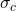=  21.84 M pa

Explanation:

Given data

Modulus of elasticity E = 225 ×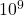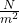Specific surface energy for magnesium oxide is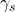= 1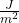Crack length (a) = 0.3 mm = 0.0003 m

Critical stress is given by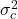=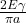——– (1)

⇒ 2 E= 2 × 225 ×× 1 = 450 ×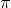a = 3.14 × 0.0003 = 0.000942

⇒ Put these values in equation 1 we get=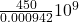= 4.77 ×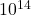= 2.184 ×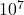=  21.84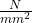=  21.84 M pa

This is the critical stress required for the propagation of an initial crack.